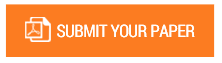Influence of Learning Model and Initial Knowledge on the Ability of Mathematic Connection

Authors : Masrifhayanti Savitri, Anton Noornia, Kadir.

Volume/Issue : Volume 3 - 2018, Issue 8 - August

Scribd : https://goo.gl/JqezHw

Thomson Reuters ResearcherID : https://goo.gl/3bkzwv

This study aims to find the effect of Problem Based Learning model and Conventional model as well as students ‘early knowledge of students’ mathematical connection ability. The research was conducted in class V SDN Rawamangun 05 Pagi East Jakarta. The method used is the experimental method with treatment design by level 2 x 2. The technique of analysis used is the analysis of two-lane variance (ANAVA). The results of this study suggest that (1) the ability of mathematical connections in groups of students who are given a problem based learning model is higher than that of the conventional modeled students, (2) there is an interaction effect between the learning model and the initial knowledge on the mathematical connection ability, the ability of mathematical connections in groups of students with high initial knowledge given the problem-based learning model is greater than the group of students given the conventional model; and (4) the students’ mathematical connection ability in the group of students with low initial knowledge given the Problem Based Learning model is lower than the student group given the conventional model.

Keywords : Problem Based Learning, conventional, preliminary knowledge, mathematical connections.

#### CALL FOR PAPERS

Paper Submission Last Date
31 - August - 2022